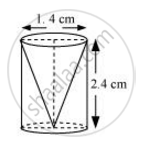Share

From a solid cylinder whose height is 2.4 cm and diameter 1.4 cm, a conical cavity of the same height and same diameter is hollowed out - CBSE Class 10 - Mathematics

ConceptSurface Area of a Combination of Solids

Question

From a solid cylinder whose height is 2.4 cm and diameter 1.4 cm, a conical cavity of the same height and same diameter is hollowed out. Find the total surface area of the remaining solid to the nearest cm[Use π = 22/7]

SolutionGiven that,

Height (h) of the conical part = Height (h) of the cylindrical part = 2.4 cm

Diameter of the cylindrical part = 1.4 cm

Therefore, radius (r) of the cylindrical part = 0.7 cm

Slant height (l) of conical part = sqrt(r^2+h^2)

= sqrt((0.7)^2+(2.4)^2) =sqrt(0.49+5.76)

=sqrt(6.25) = 2.5

Total surface area of the remaining solid will be

= CSA of cylindrical part + CSA of conical part + Area of cylindrical base

= 2πrh + πrl + πr2

= 2xx 22/7xx 0.7xx2.4+22/7xx0.7xx2.5+22/7xx0.7xx0.7

= 4.4x2.4+2.2x2.5+2.2x0.7

=10.56+5.50+1.54 = 17.60 cm2

The total surface area of the remaining solid to the nearest cm2 is 18 cm2.

Is there an error in this question or solution?

APPEARS IN

NCERT Solution for Mathematics Textbook for Class 10 (2019 to Current)
Chapter 13: Surface Areas and Volumes
Ex.13.10 | Q: 8 | Page no. 245

Video TutorialsVIEW ALL 

Solution From a solid cylinder whose height is 2.4 cm and diameter 1.4 cm, a conical cavity of the same height and same diameter is hollowed out Concept: Surface Area of a Combination of Solids.
S